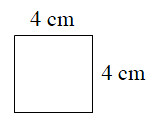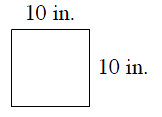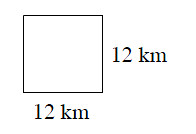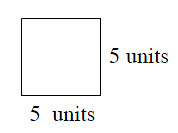### Home > MC2 > Chapter 3 > Lesson 3.1.1 > Problem3-11

3-11.

Find the area of each square with the given side lengths.

Area = base · height

(a) $16$ cm$^2$
(b) $100$ cm$^2$

1.1.1.1.1. Describe the method you used to find the area of the squares.

What did you do to find the area of each square?